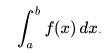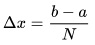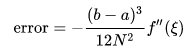# Calculating Integrals with Arduino

The Arduino platform supports math functions like sine, cosine, logarithm, exponential, etc. But can it solve higher math problems like calculus? This two-part tutorial will show you how to make a sketch for Arduino integrals and differentials solver.

### Solving Integrals Using Numerical Analysis

Integrals can be definite or indefinite. The latter one requires much more processing power than the Arduino can provide. This article will show you how to solve definite integrals only.

Numerical analysis involves the use of algorithms to solve math problems. In fact, there are algorithms for a number of complex math problems, not just integrals and differentials. Numerical analysis is what makes scientific calculators also solve calculus equations.

For this tutorial, we will be using an algorithm called trapezoidal rule to solve definite integrals. Recall that integrals are used to solve the area under the curve whose function is f(x).We can subdivide the area under the curve using trapezoids. The area of a trapezoid is

The total area will then be the sum of the areas of the trapezoid.Where Δx, sometimes called step, isOf course, the trapezoids cannot cover all the area under the curve. Numerical analysis don’t give the most accurate answer, only approximations.

The error is the difference between the actual integral and the answer given by the trapezoidal rule:,where ξ is any number between a and b and N is the number of trapezoids.

The formula above shows that the more trapezoids (N), the smaller the error becomes.

### Using Arduino to Solve Integrals

Now that we know the trapezoidal rule, we can now use it in an Arduino sketch. First, we must define what function we need to solve the integral of. Say we want to solve the integral of sinx for a given interval

The sine function is usable in an Arduino sketch through:

`sin(rad);`

We need to place this inside a function:

```double f(double x) {
sin(x);
}```

The advantage of doing this is that we can change whatever function we need the integral of in the future.

Next we define the step based on the formula given above for Δx:

`step = (upper - lower) / N;`

upper and lower here are the limits of the integral.

The integral is now then:

```integral = 0.5 * (f(lower) + f(upper));
for (int i = 0; i < N; ++i) {
integral += f(lower + step * i);
}
integral *= step;
```

The above sketch is derived from the formula above.

### Sketch for Arduino Integrals

The full sketch is now:

```double lower, upper;
int N;
double integral;
double step;
char rx_byte = 0;
String rx_str = "";
boolean done = false;

void setup(){
Serial.begin(9600);
}

void loop() {
Serial.print("Enter lower limit: ");
while(!done){
if (Serial.available() > 0) {
if (rx_byte != '\n') {
rx_str += rx_byte;
}
else {
lower = rx_str.toDouble();
done = true;
}
}
}
done = false;
Serial.print("Enter upper limit: ");
while(!done){
if (Serial.available() > 0) {
if (rx_byte != '\n') {
rx_str += rx_byte;
}
else {
upper = rx_str.toDouble();
done = true;
}
}
}
done = false;
Serial.print("Enter number of iterations: ");
while(!done){
if (Serial.available() > 0) {
if (rx_byte != '\n') {
rx_str += rx_byte;
}
else {
N = rx_str.toDouble();
done = true;
}
}
}

step = (upper - lower) / N;
integral = 0.5 * (f(lower) + f(upper));

for (int i = 0; i < N; ++i) {
integral += f(lower + step * i);
}

integral *= step;

Serial.print("Integral is equal to: ");
Serial.println(integral);

}

double f(double x) {
return sin(x);
}```

An improvement for the above sketch is to have the user give the function whose integral needs to be evaluated.

This tutorial is originally written by Roland Pelayo for circuitxcode.com